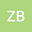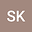Four-body quantum system in the presence of solvable potential
••• Zahra Bakhshi,
• Sare Khoshdooni
Zahra Bakhshi
Shahed University
Author ProfileSare Khoshdooni
Shahed University
Author Profile## Abstract

In this article, a four-particle quantum system is introduced with energy discrete spectrum including a harmonic potential and a three-body interaction potential. By defining the Jacobi coordinates for each particle, separately, one coordinate is eliminated as a transition in the energy spectrum. Then the system is studied in polar coordinates and by using the variables separation method, the schrödinger equation of the system is transformed into three separate differential equations. Therefore, energy eigenvalues and wave eigenfunctions are calculated in each dimension. Also, The wave eigenfunctions figures are investigated in one and three dimensions.